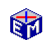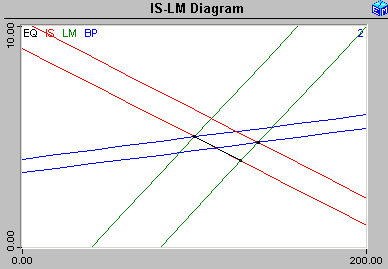The Mundell-Fleming Model

The Mundell-Fleming Model adds a balance of payments equilibrium condition (and a BP curve) to an IS-LM Model.   This extends the closed economy IS/LM framework to allow discussion of the interplay between monetary policy and exchange rate policy.  In particular, the model emphasizes the differences between fixed and floating exchange rates.

Movie:  Imperfect Capital Mobility, Fiscal Policy, Fixed Rates.
(83 seconds)

Having IS, LM, and BP curves that can intersect at one, two, or three points makes understanding the dynamics of the Mundell-Fleming Model a challenge.  This application guides you through the following steps.  By repeating the various possibilities, you will come to understand the model.Model LinkThe Mundell-Fleming Model
<
Printable PDF Exercises1.  Determine capital mobility

• Perfect Capital Mobility, Horizontal BP
• Imperfect Capital Mobility, Shallow BP
• Limited Capital Mobility, Steep BP  (steeper than LM)

2.  Find the equilibrium and draw the curves

3.  Pick a policy (choose one)

• Money Supply
• Government Spending
• Foreign Income
• Foreign Interest Rate

5.  Final Equilibrium (choose one)

• Solve Fixed Rate:  No Permanent BP Imbalance
• Solve Flexible Rate

6.  Find the equilibrium and draw the curves

Classic Economic Models

Macroeconomics

Introduction
Overview of Macro Models

Microeconomics

Introduction
Overview of Micro Models

Resources